# The Caliber¶

The caliper, also called caliper, is a measuring instrument with greater precision than a millimeter rule.

The gauge has several jaws and a probe with which outer diameters, inner diameters and depths can be easily measured.

Its vernier also called vernier scale is an auxiliary scale that allows the measurement of distances with a precision from 0.1 millimeters to 0.02 millimeters. This precision improves 10 times or more the precision of a millimeter ruler.

## Gauge parts¶

A gauge is made up of the following parts: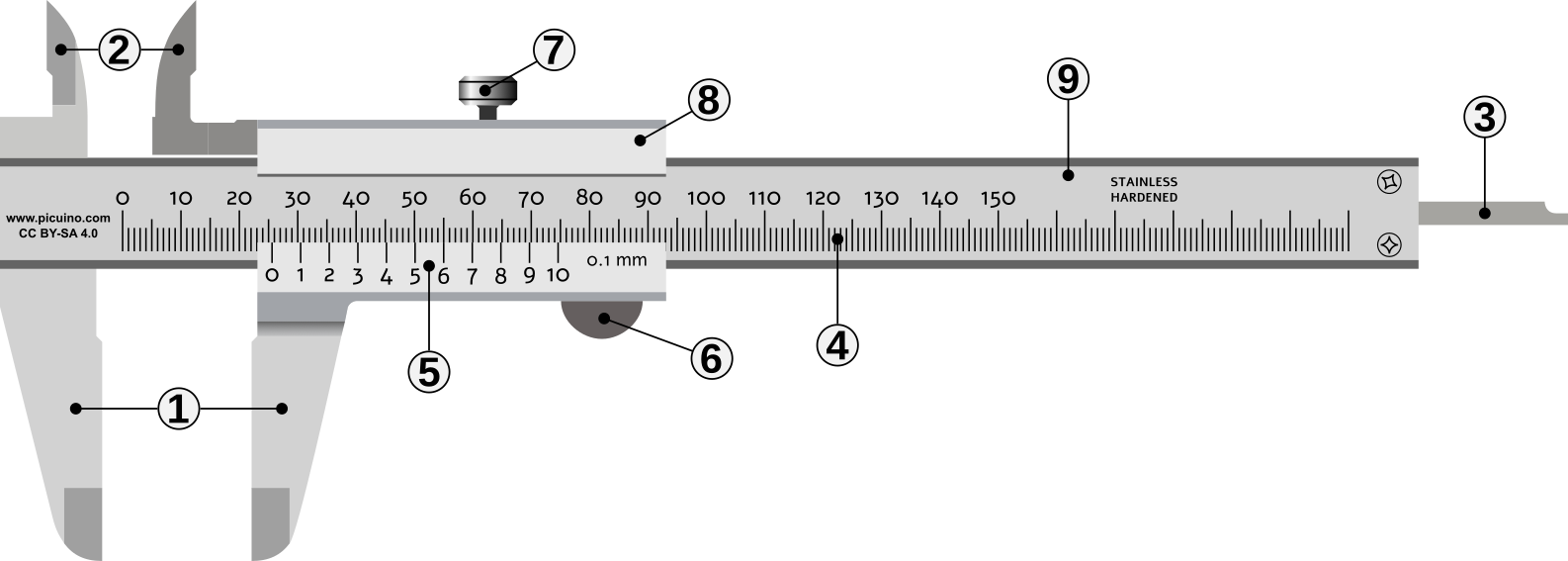Parts of a caliber or king foot.

1. External measuring jaws.
2. Internal measuring jaws.
3. Probe to measure depths.
4. Millimeter main scale.
5. Vernier to read fractions of millimeters.
6. Slide button.
7. Brake screw.
8. Slide (moving part of the gauge).
9. Rule (fixed part of the gauge).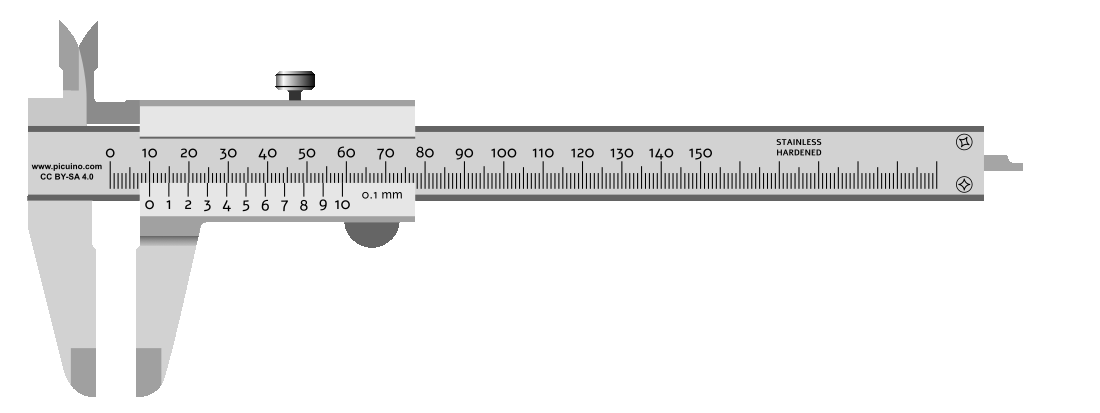One gauge slide moving on the rule.

## Carrying out measurements¶

In the following images you can see how measurements of the exterior, interior and depth of a part are taken.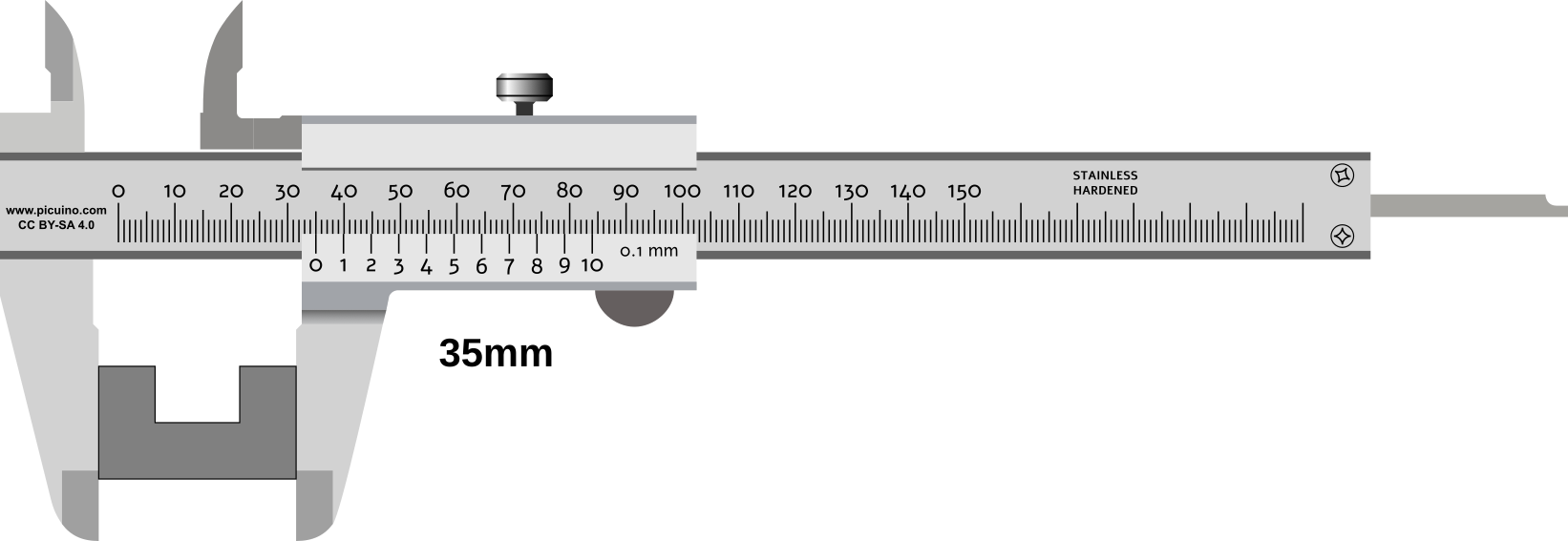Calibrate by taking an outside measurement.Calibrate by taking an inside measurement.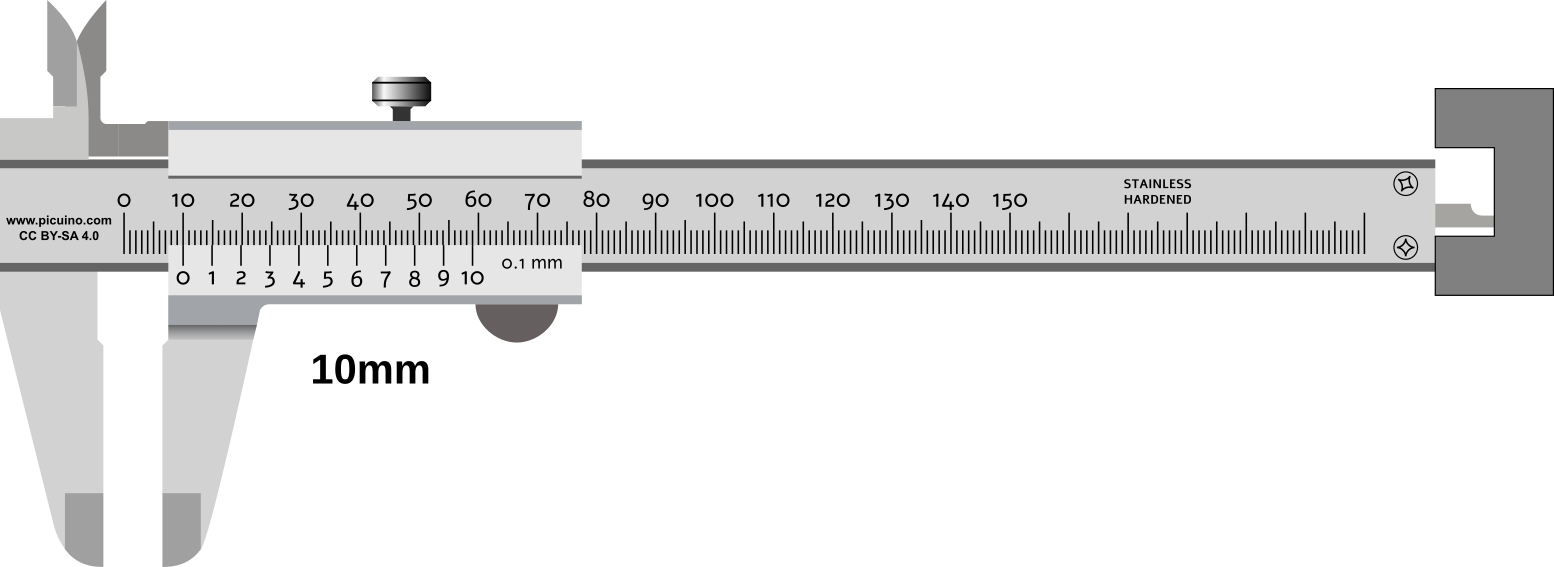Gauge by taking a depth measurement.

## Measurement with the vernier¶

The measurement in millimeters of a gauge can be seen on the main scale, where it coincides with the 0 mark of the vernier.

The measurement in tenths of a millimeter can be observed at the point where one of the lines of the vernier coincides exactly with a line of the main scale.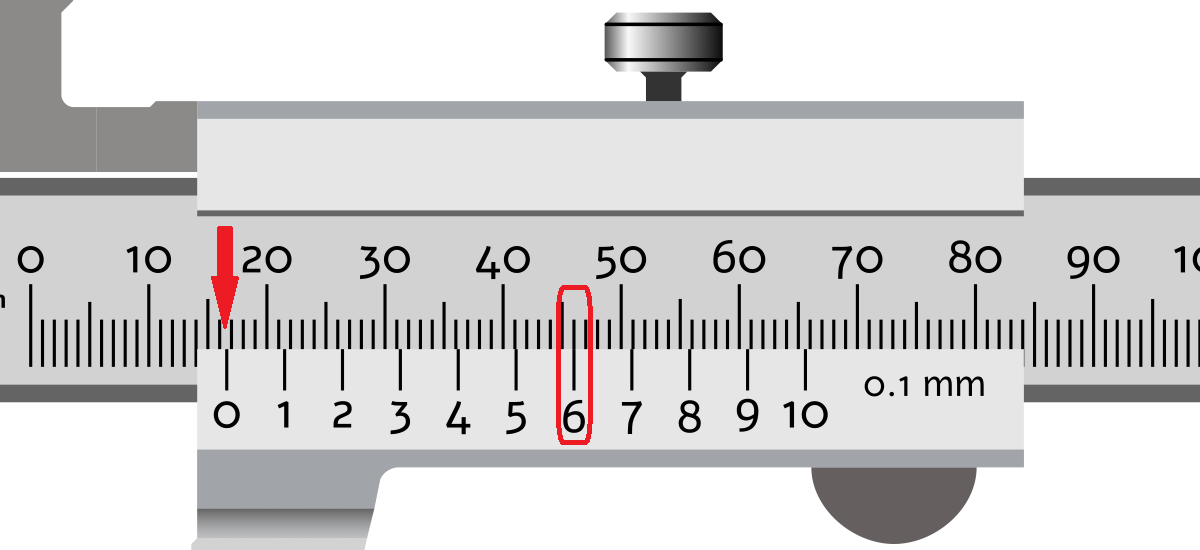Measuring a distance of 16.6 millimeters.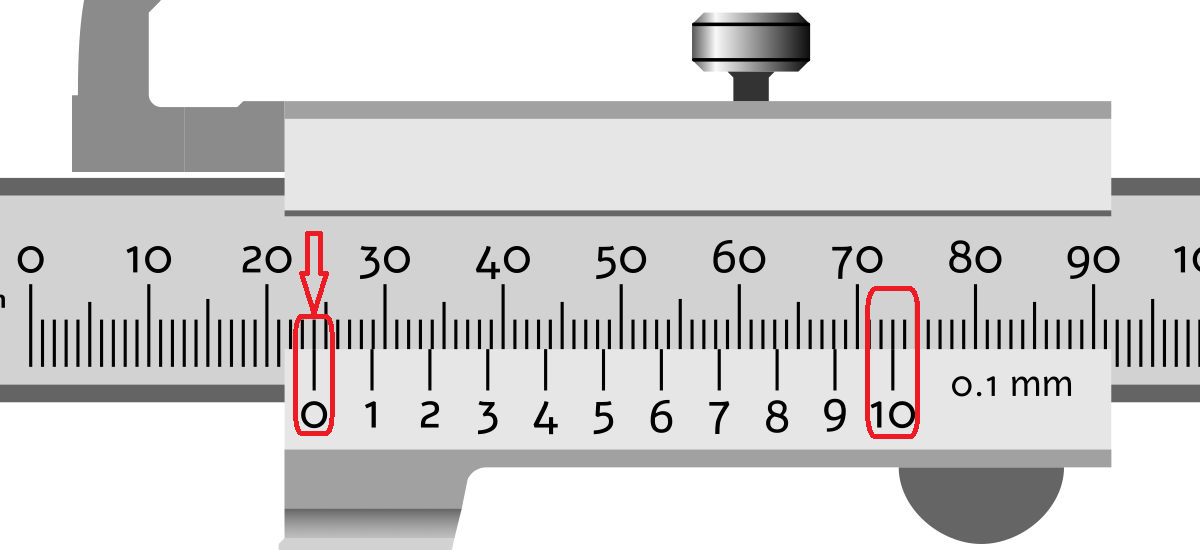Measurement of a distance of 24.0 millimeters.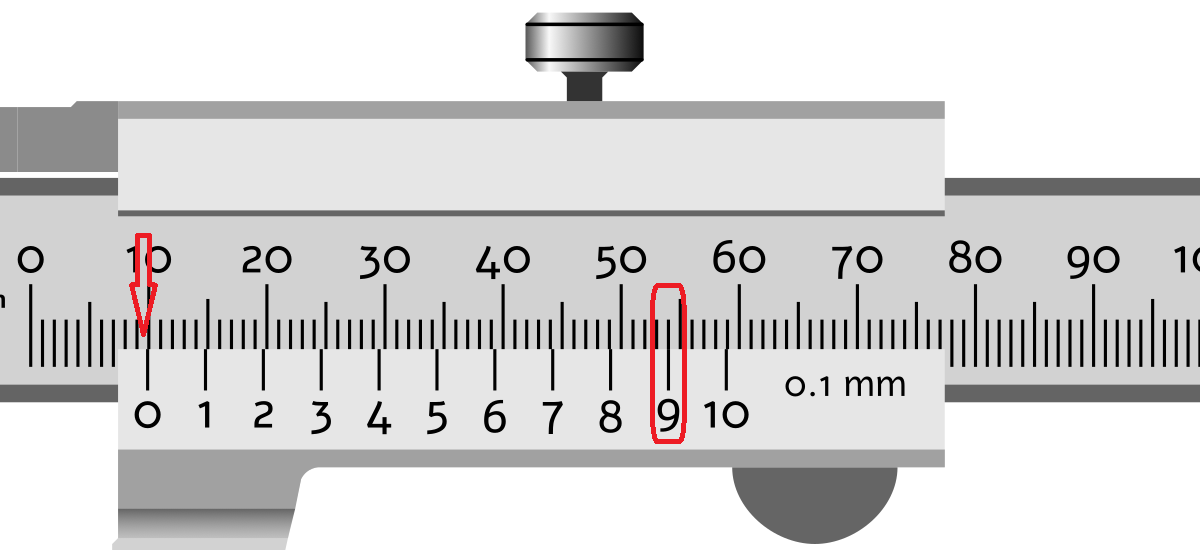Measuring a distance of 9.9 millimeters.

## Virtual gauge¶

Simulation of a caliper with a precision of 0.05 millimeters.

## Measurement exercises¶

Sheet with exercises to read distances with the caliper

`Exercises of measurements with caliber. PDF format.`

## Unit test¶

Online measurement test with caliber.